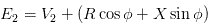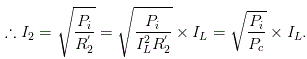# MCQs on Electrical Machines

##### Page 103 of 114. Go to page 1 2 3 4 5 6 7 8 9 10 11 12 13 14 15 16 17 18 19 20 21 22 23 24 25 26 27 28 29 30 31 32 33 34 35 36 37 38 39 40 41 42 43 44 45 46 47 48 49 50 51 52 53 54 55 56 57 58 59 60 61 62 63 64 65 66 67 68 69 70 71 72 73 74 75 76 77 78 79 80 81 82 83 84 85 86 87 88 89 90 91 92 93 94 95 96 97 98 99 100 101 102 103 104 105 106 107 108 109 110 111 112 113 114
01․ If in a sub Ã¢â‚¬â€œ station there is one 132 / 33 kV transformer whose secondary is connected with primary of one 33 / 11 kV transformer, the total transformation ratio of the sub Ã¢â‚¬â€œ station will be,
7.
12.
4.
3.

If two transformers are connected in such a way that secondary of first one is connected with primary of second, and if transformation ratios of these two transformers are K1 and K2, total or overall transformation ratio of this system will be K1K2.

02․ A short circuit test on a transformer gives

In a short circuit test we apply variable voltage at HV terminals of a transformer keeping LV side short circuited. The applied voltage is varied until the input current reaches its rated value or full load value. As the current drawn from source is full load current the test normally measures the copper losses of transformer at full load. But since, by adjusting variable supply voltage we can control the supply current at any value, a short circuit test can give copper loss at any load.

03․ A step up transformer increases
power.
voltage.
current.
frequency.

A step up electrical transformer transforms a power from one circuit to another without changing its frequency to higher voltage level and proportionate lower current level.

04․ In a step down transformer which one of the following statements is true?
Number of secondary turns is less than primary turns.
Secondary power is less than primary power.
Secondary current is less than primary current.
Number of secondary turns is more than that of primary.

In a transformer voltage per turn is same in both primary and secondary winding hence, induced voltage across the winding is proportional to number of turns in the winding. As in step down transformer secondary voltage is less than primary, the number of turns in secondary is obviously less than that of primary.

05․ When a transformer is loaded, the secondary terminal voltage drops. That means power factor is
zero.
unity.
lagging.

Approximate relation of no load and full load voltages in a transformer is,Where E2, V2, R, X and cosθ  are no load voltage, full load voltage, secondary resistance of transformer, secondary leakage reactance of transformer and power factor respectively. If power factor is lagging, θ  is positive which means ( Rcosθ  + Xsinθ ) > 0. Thus E2 > V2.

06․ If Kh is hysteresis constant, f is supply frequency, Bm maximum flux density in core of transformer, the expression of hysteresis loss in transformer, is given by
KhfBm1.6.
KhfBm3.
Khf2Bm1.6.
KhfBm.

If Kh is hysteresis constant, f is supply frequency, Bm maximum flux density in core of transformer, the expression of hysteresis loss in transformer is given by KhfBmx watts. Where x ranges from 1.5 to 2.5.

07․ If Ke is eddy current constant, Kf is form factor, f is supply frequency, Bm maximum flux density in core of transformer, the expression of eddy current loss in transformer, is given by
Kef2KfBm2.
KefKf2Bm2.
Kef2Kf2Bm2.
Kef2Kf2Bm.

If Ke is eddy current constant, Kf is form factor, f is supply frequency, Bm maximum flux density in core of transformer, the expression of eddy current loss in transformer is given by Kef2Kf2Bm2.

08․ Stray losses in transformer occurs in
steel core.
winding conductors and steel structures of transformer.
transformer insulating oil.
paper insulation in transformers.

Stray loss occurs in the mechanical structures and winding conductors due to stray fluxes.

09․ If iron loss and full load copper loss of any transformer are denoted by Pi and Pc, then the load corresponding to maximum efficiency is given by
(Pi / Pc)0.5 Ãƒâ€” Full Load.
(Pi / Pc)2 Ãƒâ€” full Load.
(Pi / Pc) Ãƒâ€” full Load.
(Pi / Pc)1.6 Ãƒâ€” full Load.

At maximum efficiency of transformer, iron-loss = copper-loss e.i. Pi = I22R2. Where I2 is load current at maximum efficiency and R2 resistance of transformer referred to secondary.Where IL is full load current of transformer and R2 is the equivalent resistance of the transformer referred to load side.

10․ Secondary winding of an auto transformer is also called
compensating winding.
common winding.
tertiary winding.
damping winding.

The auto transformer has a single continuous winding which is used for both input and output voltages. Secondary winding of auto transformer carries both the supply current and load current. But these two currents are practically in phase opposition. Hence secondary winding of auto transformer is also called common winding.

<<<101102103104105>>>